## Lesson 5.1 The basics

### Video: 5.101 Introduction to Boolean algebra

• Boolean Algebra
• History
• 384-322 BC: Aristotle develops the foundations of logic.
• 1854: George Boole published An investigation of the laws of thought
• 1904: H.E. Huntington wrote Sets of independent postulates for the algebra of logic.
• 1938: Claude Shannon wrote a thesis: A symbolic analysis of relay switching
• Boolean algebra is the foundation of computer circuit analysis.
• Basic building block for designing transistors, basic elements in processors.
• Consider IoT fire system: when high heat is detected, spray water.
• Two-valued Boolean Algebra
• Most well-known form of Boolean algebra is 2 valued system:
• variables take value in set {0, 1}
• operators + and . correspond to OR and AND.
• Used to describe and design digital circuits.
• Operations of Boolean Algebra
• Based on 3 fundamental operations:
• AND
• logical product, intersection or conjunction
• represented as x . y, $x \cap y$ or $x \wedge y$.
• OR
• logical sum, union or disjunction
• represented as $x + y$, $x \cup y$, $x \lor y$
• NOT
• logical complement or negation
• represented as $x'$, $\overline{x}$ or $\neg x$
• When parentheses are not used, orperators have order of prefs: NOT > AND > OR.
• Operations of Boolean algebra
• The truth tables for the 3 operations can be represented as follows: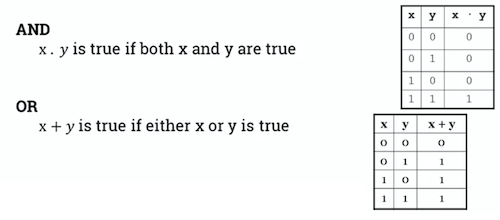### 5.103 Postulates of Boolean algebra

• Huntington's Postulates
• Huntington's posulates define 6 axioms that must be satisfied by any Boolean algebra:
• closure with respect to the operators:
• any result of logical operation belongs to the set {0, 1}
• identity eleements with respect to the operators:
• $x + 0 = x$
• $x \ . \ 1 = x$
• commutativity with respect to the operators (dot and .):
• $x + y = y + x$
• $x . y = y . x$
• distributivity:
• $x(y + z) = (x . y) + (x . z)$
• $x + (y . z) = (x + y) . (x + z)$
• commutativity
• $x + y = y + x$
• $x . y = y. x$
• distributivity
• $x(y + z) = (x . y) + (x . z)$
• $x + (y . z) = (x + y) . (x + z)$
• complements exist for all elements
• $x + x' = 1$
• $x . x' = 0$
• distinct elements
• $0 \ne 1$
• Basic Theorems of Boolean Algebra

• Using the 6 axioms of Boolean algebra, we can find these useful theorems for analysing and designing circuits
• theorem 1: idempotent laws
• $x + x = x$
• $x . x = x$
• theorem 2: tautology and contradiction
• $x + 1 = 1$
• $x . 0 = 0$
• theorem 3: involution
• $(x')' = x$
• theorem 4: associative laws
• $(x + y) +z = x + (y + z)$
• $(x . y) . z = x . (y . z)$
• theorem 5: absorption laws
• $x + (x . y) = x$
• $x . (x + y) = x$
• theorem 6: uniqueness of complement
• if $y + x = 1$ and $y . x = 0$ then $x = y'$
• theorem 7: inversion law
• $0' = 1$, $1' = 0$
• De Morgan's Theorems

• Theorem 1
• The complement of a product of variables is equal to the sum of the complements of the variables: $\overline{x. y} = \overline{x} + \overline{y}$
• Theorem 2

• The complement of a sum of variables is equal to the product of the complements of the variables: $\overline{x + y} = \overline{x} . \overline{y}$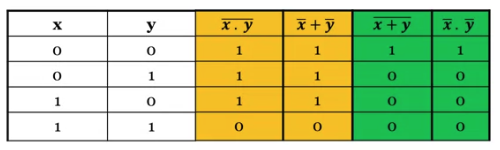• Proof of distributivity of + over .

• A truth table to prove the distributivity of + over . using truth tables.• Principle of duality
• Starting with a Boolean relation, we can build another equivalent Boolean relation by:
• changing each OR (+) sign to an AND (.) sign
• changing each AND (.) sign to an OR (+) sign.
• changing each 0 to 1 and each 1 to 0.
• Example
• Since A + BC = (A + B)(A + C) (by distributive law), we can build another relation using the duality principle: $A(B + C) = AB + AC$
• Examples
• Consider the boolean equations:
• $e1: (a . 1) . (0 + \overline{a}) = 0$
• e2: $a + \overline{a} . b = a + b$
• Dual equations of e1 and e2:
• Dual of e1: $(a + 0) + (1 . \overline{a}) = 1$
• Dual of e2: $a . (\overline{a} + b) = a . b$
• Ways of proving theorems
• 4 ways in general to prove equivalence of Boolean relations:
• perfect induction by showing 2 expressions have identical truth tables: tedious if more than 2 vars.
• axiomatic proof by applying Huntington's postulates or theorems to the expressions, until identical expressions are found.
• duality principle every theorem in Boolean algebra remains valid if we interchange all ANDs and ORs and interchange all 0s and 1s.
• contradiction by assuming the hypothesis is false then proviing that the conclusion is false.
• Examples
• Proving absorption theorem
• The absorption theorem can be proved using perfect induction, by writing a truth table.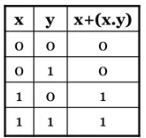• It can also be proved directly:
• From $x + (x . y) = x$, if we apply the duality principle, we can deduce: $x . (x + y) = x$

\begin{align} x + (x . y) &= (x . 1) + (x . y) \text{ by } x .1 = x \\ &= x . (1 + y) \text{ by distributivity } \\ &= x . (y + 1) \text{ by commutativity } \\ &= x . 1 \text{ by } y + 1 = 1 \\ &= x \text{ by } x.1 = x \\ \end{align}

### 5.105 Boolean functions

• Boolean Function
• A boolean function defines a mapping from one or multiple Boolean input values to a Boolean output value.
• For $n$ Boolean input values, there are $2^n$ possible combinations.
• For example, a 3-input function $f$ can be completely defined with an 8-row truth table.
• Algebraic forms
• There is only one way to represent a Boolean function in a truth table.• In algebraic form, a function can be expressed in a variety of ways:
• For example these are both algebraic representations of the same truth table:
• $f(x) = x + x' . y$
• $f(x) = x + y$
• Standardised forms of a function
• The two most common standardised forms:
• sum-of-products form
• product-of-sums form
• Sum-of-Products Form:
• Variables built using the AND operator, are summed together using the OR operator.
• Example: $f(x, y, z) = xy + xz + yz$
• Product-of-Sums Form
• Variables built using the OR operator, are multiplied together using the AND operator.
• Example: $f(x, y, z) = (x + y)(x + z)(y + z)$
• The sum-of-products form is easier to use so it's used by the course.
• Building a sum-of-products form
1. Focus on the values of the variable that make the function equal to $1$.
1. If an input equals $1$, it appear uncomplemented in the expression.
1. If an input equals 0, it appears complemented in the expression (and its corresponding complete is used).
1. the function f is then expressed as the sum of products of all the terms for which f = 1
• Example
• Let's consider the function f represented by the following truth trable.
• Can be expressed as:
• $f(x, y) = x ' y + xy' + xy$
• Useful functions
• The "exclusive-or" function: $x \oplus y$:
• defined as "true if either x or y is true, but not both"
• represented by this truth table: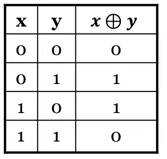• can be expressed as:
• $x \oplus y = x ' y + xy'$
• The "implies" function: $x \rightarrow y$:
• defined as "if x then y"
• represented by this truth table: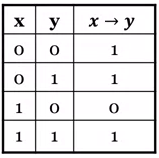• can be expressed as:
• $x \rightarrow y = x' + y$